Courses

IIFT Quantitative Ability MCQ Quiz - 2

22 Questions MCQ Test IIFT Mock Test Series | IIFT Quantitative Ability MCQ Quiz - 2

Description
Attempt IIFT Quantitative Ability MCQ Quiz - 2 | 22 questions in 20 minutes | Mock test for CAT preparation | Free important questions MCQ to study IIFT Mock Test Series for CAT Exam | Download free PDF with solutions
QUESTION: 1

Consider set S= {80, 30, 42, 10, 13, 66, 99, 40}. If two two-digit numbers x andy are added to the set (x < y) such that all the elements of this set are distinct, what is the difference between the maximum and minimum possible value of the median of the new set?

Solution:

S (in ascending order) = {10, 13, 30, 40, 42, 66, 80, 99}

the median of the series  = (40+42)/2= 41

Now, x andy are to be added to this series. This can be added in the following ways:

(i) To get the minimum possible value of the median, x and y have to be as small as possible, since they are both two digit numbers, consider the following values for x and y:

x = 11 andy = 12

S" = {10, x, y, 13,30,40,42, 66, 80, 99}

Median = (30+40)/2 = 35

(ii) To get the maximum possible value of the median, x andy have to be as big as possible, since they are both two digit numbers, consider the following values for x and y:

x = 97 andy = 98

S"= {10, 13,30, 40, 42, 66, 80,x,y, 99}

Median = (42+66)/2 = 54

∴ The difference between the maximum and minimum possible values of the new median = 54 - 35 = 19

Hence, option 1.

QUESTION: 2

Two children are given ropes of the same length. They both make geometric figures without any wastage of rope. One child makes an equilateral triangle while the other makes an isosceles right angled triangle. If the area of the isosceles right angled triangle is 8 cm2, what is the approximate length of rope per side that goes into making the equilateral triangle?

Solution:

Since the two children are given ropes of the same length, and there is no wastage in making the two figures, the two triangles have the same perimeter.

Let the congruent sides of the isosceles right triangle be x cm.

∴ Perimeter of isosceles right triangle = x + x +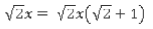Also, area  of isosceles right triangle =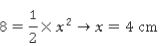∴ Perimeter of isosceles right triangle =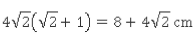This is the same as the perimeter of the equilateral triangle.

∴ Side of equilateral triangle =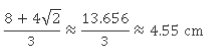Hence, option 1.

QUESTION: 3

A man deposits Re.l in a bank. The annual nominal interest rate is 10% per annum. The man is free to choose the time period after which he wants to compound the interest (i.e. he can choose the number of times the interest is compounded per year). What is the maximum amount he can receive at the end of two years? Take e01 = 1.1051 if you need it.

Solution:

Let the interest be compounded n times a year. Hence, the man will get a return of;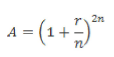Here, r is the nominal interest rate (as a decimal) and time period is 2 years.

Now, if the compounding is done once a year, amount = (1 + 1/10)2 = Rs. 1.21
If the compounding is done every six months, then amount = (1 + 1/20)4 = Rs. 1.2155

If the compounding is done every three months, then amount = (1 + 1/40)8 = Rs. 1.2184

So, we get the maximum return if the interest is calculated every instant (i.e. n —> ∞).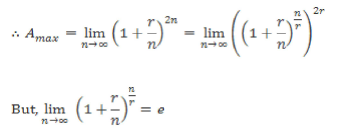Hence, Amax = e2r= e2x0.1 = e02= (1.1051)2 = 1.2212

Hence, option 1.

QUESTION: 4

Sum of first 35 natural numbers, when one two-digit number is added wrongly as its reverse, consequently causing another to be added twice, comes out to be 648. Find the number which is added wrongly as its reverse.

Solution:

Sum of first 35 natural numbers = (35 x 36)/2 = 630, but the sum given is

∴ The wrong number is (648 - 630) = 18 more than the number that is added twice.
Also, as the wrong number is added twice, it has to be less than 35.
The only such number is 13.
Hence, option 1.

QUESTION: 5

A merchant can buy goods at the rate of Rs. 20 per good. The particular good is part of an overall collection and the value is linked to the number of items that are already on the market. So, the merchant sells the first good for Rs. 2, second one for Rs. 4, third for Rs. 6…and so on. If he wants to make an overall profit of at least 40%, what is the minimum number of goods he should sell?

Solution:

Let us assume he buys n goods.

Total CP = 20n

Total SP = 2 + 4 + 6 + 8 ….n terms

Total SP should be at least 40% more than total CP

2 + 4 + 6 + 8 ….n terms ≥ 1.4 * 20 n

2 (1 + 2 + 3 + ….n terms) ≥ 28n

n(n + 1) ≥ 28n

n2 + n ≥ 28n

n2 - 27n ≥ 0

n ≥ 27

QUESTION: 6

A bird, which is flying at a constant height from the ground, makes an angle of depression of 45° with the bottom of a pole and 30° with the top of the pole. When the bird is directly above the pole, what is its approximate elevation from the top of the pole, given that the height of the pole is 8 m?

Solution:

Let the height of the bird from the top of the pole be x.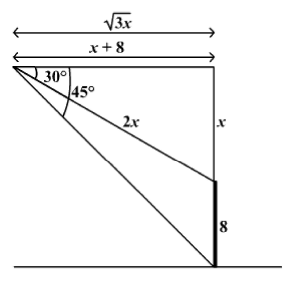As shown in the figure and using the properties of 30-60-90 and 45-45-90 triangles,

√3x = x + 8

∴ x = 10.92 m ~ 11 m

Hence, option 2.

QUESTION: 7

Some money is distributed among four brothers in such a way that the amount received by them is in the ratio 6 : 7 : 8 : 9 (in descending order of age). Also, if the amount received by each brother is squared and then added, the amount becomes 5750. How much money have the three elder brothers received in all?

Solution:

Let the brothers receive Rs. 6x, Rs. 7x, Rs. 8x and Rs. 9x respectively.

∴ 36x2 + 49x2 + 64x2 + 8lx2 = 5750 ∴ 230x2 = 5750

∴ x2 = 25

∴x = 5
∴ Amount received by the three elder brothers = 6x + 7x + 8x = 2 lx = Rs. 105
Hence, option 1.

QUESTION: 8

An industrial facility gave the cost incurred for production of x units of a particular product as C = 470x - 41x2 + x3 and the warehouse gave the revenue generated from the sale of the products as a S' = 320x + 115x2. What is the output from the warehouse at which the average profit from the product is the maximum?

Solution:

Profit from x units of the product is P = S - C = 156x2 - 150x - x3

Average profit = f[x) = P/x = 156x - 150 - x2

Now,f(x) = 156 - 2x

f"' ( x ) = - 2

Since, f'(x) is negative, function f(x) assumes maximum value at the value of x corresponding to f ( x ) = ∴ 156 - 2x = 0

i.e. x = 78

Hence, option 2.

QUESTION: 9

Thirteen caps shaped like a right circular cone are to be distributed to kids for a birthday party. Each cap has to have a base radius of 7.5 cm and height of 10 cm. What is the area of cardboard required to make the caps, assuming no cardboard is wasted?

Solution:

Since the caps are shaped as a right circular cone and 13 such caps are needed,

Total area of cardboard required = 13  curved surface area of one cap

=13πrl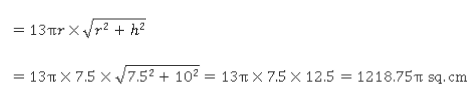Hence, option 1.

QUESTION: 10

If a + b + c — 11 what is the maximum value of (a — 2)(b — 1 )(c +1) for natural values of a ,b, c ?

Solution:

if x + y + z is constant, the product xyz takes its maximum value when x = y =z

QUESTION: 11

The speed of a motorboat in still water varies with the number of people on it as follows: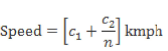where c1and c2 are constants and n is the number of people on the motorboat.The speed of the boat when there is only one person on board is 50 kmph and 35 kmph when there are four people on board. On one particular day, the motorboat starts from Aksa beach to an island which is 500 kms away with the driver and one passenger on board. Immediately after reaching the island, no one gets off and the boat starts for its return journey with three more passengers on board. The current of the sea water is 10 kmph away from the island towards Aksa beach. Finally, the boat reaches Aksa beach. Find the average speed for the entire ioumey.

Solution:

The speed of the boat when there is only one person on board is 50 kmph.

∴ c1 + c2 — 50 ... (1)

The speed of the boat when there are 4 people on board is 35 kmph.

∴ c1+ (c2/4) = 35 ... (2)

On solving (1) and (2), c1= 30 and c2 = 20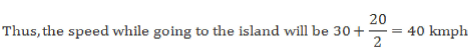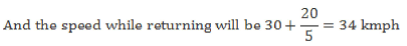The current of the sea water is 10 kmph away from the island and towards Aksa beach.

Thus, the effective speed while going to the island will be 40 - 10 = 30 kmph and the effective speed while returning will be 34 + 10 = 44 kmph.

Since the same distance is travelled in both parts of the journey, the average speed will be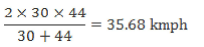Hence, option 3.

QUESTION: 12

Two concentric circles with integral radii are drawn with centre at point C. A chord PQ, 14 units in length, to the outer circle is also a tangent to the inner circle. What is the sum of the diameters of the two concentric circles?

Solution: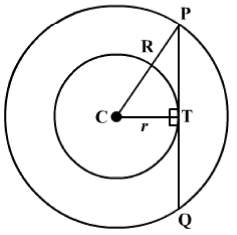The given data could be represented as shown in the above diagram.

Let R and r denote the radii of the outer and the inner circle respectively.
PQ is a tangent to the inner circle, hence it is perpendicular to the line joining the centre (C) of the inner circle and the point of intersection (T) of the circle and the tangent. Here, PT = TQ = 7

Also, ∠CTP =∠CTQ = 90°

In ΔCTP, CP2 = CT2 + PT2

R2 = r2 + 72

Thus, 7, r and R form a Pythagorean triple. 7 is part of only one such triplet, 7, 24 and 25.

r = 24,R = 25

Sum of the radii of both the circles = r + R = 24 + 25 = 49

The sum of the diameters of both the circles = 2 x 49 = 98

Hence, option 1.

QUESTION: 13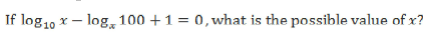Solution: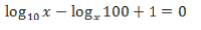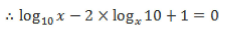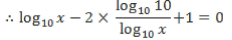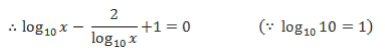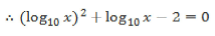Let log10x = y

∴ y2+ y - 2 = 0

∴( x+ 2)(y - 1) = 0

∴ y = 1 or -2

For, y = log10 x= 1, x = 10

Hence, option 3.

QUESTION: 14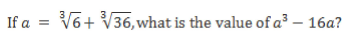Solution: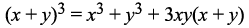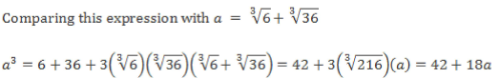∴ a 3 - 1 6 a = 42 + 1 8 a - 1 6 a = 42 + 2 a

Hence, option 3.

QUESTION: 15

John, Tom and Harry completed an assignment by working for a total of 60 days. During this period, each one of them remained absent for some days. John remained absent for 20 more days than the number of days for which Tom remained absent. When the assignment got completed, it was found that Harry completed only one third of the total work. For how many days more did Harry work than John?

Solution:

As nothing is stated otherwise, we can assume that each of them contributed the same amount of work on a day when he was present at work. Let this amount of work be k. Further, let the number of days John, Tom and Harry remained present at work be (x - 20), x and y respectively. We have: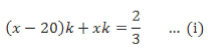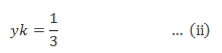Simplifying, we gety = x - 10

∴The number of days for which Harry worked more than John = y — (x - 20) = (x - 10) - (x - 20) = 10 days.

Hence, option 2.

QUESTION: 16

Joining the mid points of a regular hexagon of side a units a second hexagon is formed. Again a third hexagon is formed by joining the mid points of the second hexagon. This process is repeated infinite number of times. What is the sum of areas of all the hexagons so formed?

Solution: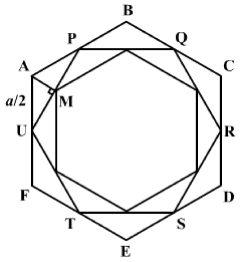Area of the outermost hexagon ABCDEF =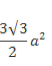As shown in the figure infinite number of hexagons is drawn.

Draw perpendicular AM on side UP.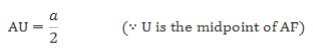Since m∠UAP = 120° and AM is the perpendicular bisector, Δ AMU is a 30- 60-90 triangle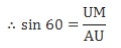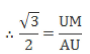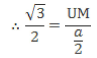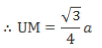UP — 2UM — side of the second hexagon =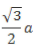Area of the second hexagon PQRSTU =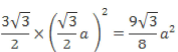∴ A rea o f th e second hexagon is 3/4tim es th a t o f th e first

∴ Similarly , area of the third hexa gon is 3/4 times  that of  the  seco nd and  so on,

∴ The areas of all the inner hexagons form an infinite decreasing G.P.

Com m on ratio (r) 3/4 nd first term (a )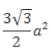∴ Sum of the are as  of  all  the  hexagons =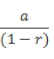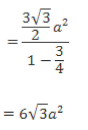Hence, option 2.

QUESTION: 17

When 746 x 321 is divided by 25, the result is M and {M} denotes its fractional part. If the fractional part is of the form (0 .yd), what is the value of dl

Solution: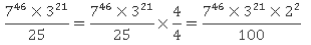Unit’s digit of 746 = Units digit of 72 = 9

Unit’s digit of 321 = Units digit of 31 = 3

Unit’s digit of 22 = 4

∴ U nit’s digit o f 746 x 321 x 22 = units digit of  9 x 3 x 4 = 8

Thus, the given term is of the form abcd... efgh... 8.

When this term is divided by 100, the outcome is of the form (abed.. .efgh..) + 0.yS

∴  d= 8

Hence, option 1.

QUESTION: 18

If abc is a three digit number such that the product of abc with the sum of its digits is 2005, what is bl

Solution:

Product of abc and the sum of its digits is 2005.

∴ (100a + 10 b + c){a + b + c) = 2005

Now, either the number should have its units digit as 5 or the sum of its digits should have its units digits as 5, or both.

Hence, try to express 2005 as a product of two numbers such that at least one of the two has its units digit as 5 and one of the two numbers is a 3-digit number.

2005 = 401 x 5

Since 5 and 401 are both prime numbers, there is no other way of expressing 2005 in the manner required.

∴ abc = 401

∴ b = 0

Hence, option 4.

QUESTION: 19

A king has hundred sons. He takes every ten sons to a jungle for hunting with no ten being repeated.
Let X be the number of times that the kings goes to the forest and 7 be the number of times that each son goes to the jungle.
F i n d X -Y.

Solution:

Given that the king takes every ten of his hundred sons to the jungle with no ten being repeated

∴ The number of times that the king goes to the forest is given by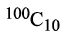i-e. X =Also, each son goes with every two of his brothers selected from the remaining 6 brothers.

∴ The number of times that each son goes to the forest is given by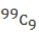i.e. Y =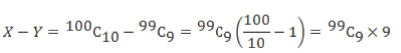Hence, option 4.

QUESTION: 20

We have two motorcyclists cycling in opposite directions at speeds of 33 m/s and 44 m/s respectively on a circular track of radius 105 m, starting from the same point. What is the time taken by the motorcyclists to meet for the fifth time at the starting point?

Solution:

Circumference of the track = Distance that has to be travelled by both the motorcycles to complete one round = 2 x 22/7 x 105 = 660 m.

Time taken by the first motorcycle to complete one round = 660/33 = 20 seconds

Time taken by the second motorcycle to complete one round = 660/44 = 15 seconds

∴ The two motorcycles meet for the first time at the starting point at LCM (15, 20) = 60 seconds

So, they meet for the fifth time at 60 x 5 = 300 seconds = 5 minutes.

Hence, option 4.

QUESTION: 21

From a deck of 52 playing cards, a card is picked and checked if it is a queen or not. This is done 15 times with replacement. What is the probability of drawing a queen exactly 4 times?

Solution:

Probability of drawing a queen = 4/52

Probability of drawing any card other than the queen = 48/52

∴ Probability of drawing a queen exactly 4 times out of 15

=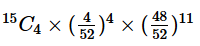QUESTION: 22

The time taken to travel from Mumbai to Pune varies directly as the distance and inversely as the velocity of the train. The velocity varies directly as the square root of the number of units of electricity used per km and inversely as the number of coaches attached to the engine. It takes three hours to reach Pune when 15 coaches are attached to the engine and 2025 units of electricity are utilized per km. What should be the number of electricity units utilized per km in order to reach Pune in the same time if 20 coaches are attached to the engine?

Solution:

Let t be the time taken, d be the distance, TV be the number of coaches and U be the number of electricity units utilized per km. Let k be the constant of proportionality.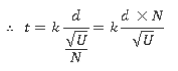In the first case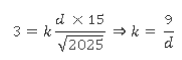Now, in the second case, N= 20 and t = 3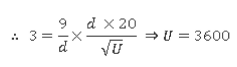Hence, option 3.Use Code STAYHOME200 and get INR 200 additional OFF Use Coupon Code

How to Prepare for CAT

Read our guide to prepare for CAT which is created by Toppers & the best Teachers

Track your progress, build streaks, highlight & save important lessons and more!

Similar ContentRelated tests